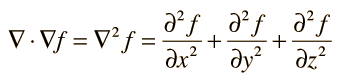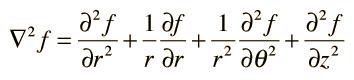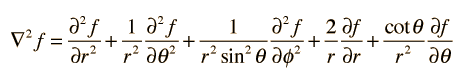The LaPlacian

The divergence of the gradient of a scalar function is called the Laplacian. In rectangular coordinates:The Laplacian finds application in the Schrodinger equation in quantum mechanics. In electrostatics, it is a part of LaPlace's equation and Poisson's equation for relating electric potential to charge density.

LaPlacian in other coordinate systems

Index

Vector calculus

 HyperPhysics*****HyperMath*****Calculus R Nave
Go Back

Laplacian, Various Coordinates

Compared to the LaPlacian in rectangular coordinates:In cylindrical polar coordinates:and in spherical polar coordinates:Index

Vector calculus

 HyperPhysics*****HyperMath*****Calculus R Nave
Go Back